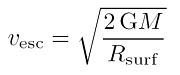# What is the value of the escape speed

## Escape speed

The escape speed or Escape speed is the speed that a body has to reach in order to escape the gravitational field of a mass.

### A little billThe frame dragging of the means of Newtonian gravitational physics can be done quickly by equating the kinetic energy with the potential energy of a test mass in the gravitational field of a large mass M. the escape speed vesc calculate (result on the right). The gravitational field is assumed to be spherically symmetrical and the smaller test mass at location R.surfe.g. the surface of the great mass M., localized. G is Newton's gravitational constant with the numerical value 6.672 × 10-11 m3 kg-1 s-2 in SI units.

### Examples

The speed of escape from the earth is 11.2 km / s or a good 40,000 km / h. That is quite a decent speed that rockets have to achieve in order, for example, to bring satellites into Earth orbit.
The extreme is a black hole in these considerations: here the escape speed corresponds to the speed of light in a vacuum c, a whopping 300,000 km / s or 1.08 billion km / h! According to the special theory of relativity is c however, the general top speed. Only light can achieve this 300,000 km / s, but not matter. In other words, not even light can escape a black hole at a critical distance, the event horizon. Therefore, when viewed from the outside, the hole is black.

### further names

The escape speed will too parabolic speed called because the escaping body escapes on a parabolic path. Another name for the escape speed issecond cosmic speed.
Annotation: The first cosmic speed is the minimum speed that a body must have in order to orbit a mass on a circular path; it is less than the escape speed. Upon reaching the third cosmic speed the body also leaves the mass, but on a hyperbolic path (therefore also hyperbolic speed); it is even greater than the escape speed.

### Limits of Newton's Theory

It should be noted that with such a compact mass as a black hole it cannot be calculated with the means of Newtonian gravitational physics. Here begins the regime of a new theory of gravity, namely Einstein's General Theory of Relativity (GTR). She understands gravitation geometrically as a curved space-time. It is a coincidence that the equation above still produces the correct result, namely the so-called Schwarzschild radius; thus Newton's gravity fails in the analog calculation for the case of a rotating black hole. This is correctly described by the Kerr solution of ART.